# Formulas and Functions

• In excel, A Formula is an expression which calculates the value of a cell. For example, =A1+A2+A3, which finds the sum of the range of values from cell A1 to Cell A3.
• Functions are predefined formulas in Excel.
>> Cell A4 below contains a formula which adds the value of cell A1 , A2 & A3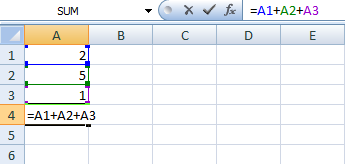>> Cell A4, below contains the SUM function which calculates the sum of the range A1:A3.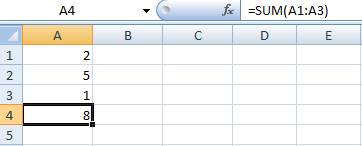### How to Enter a Formula

To enter a formula, take following steps
>> Select a cell.
>> To let Excel know that you want to enter a formula, type an equal sign (=).
>> and type the formula A1+A2+A3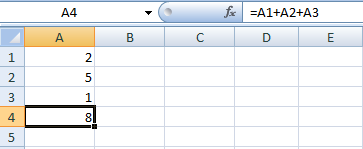There's a shortcut too..
>> instead of typing A1 , A2 & A3 , u can select cell A1 , A2 & A3.
• Now change the value of cell A1 to 5.
• Excel will automatically calculate the value of cell A4.
• Likewise the cell A4 value be recalculated if you change any value from cell A1 to cell A3.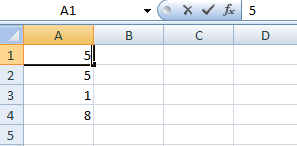.

### How to edit a Formula

When you select a cell, Excel shows formula of the cell in the formula bar.Simple steps to edit a formula
>> select the cell containing the formula you want to edit
>> click in the formula bar to edit formula
>> change the formula.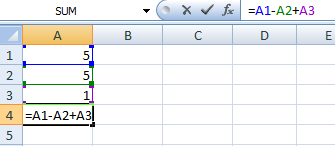>> Press Enter.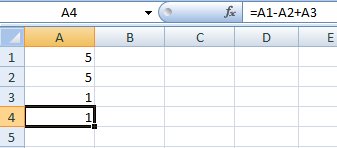### Operator Precedence

Excel calculates in a default order. i.e if you combine several operators in a single formula, Excel performs the operations in the order shown in the following table. If a formula contains operators with the same precedence — for example, if a formula contains both a multiplication and division operator — Excel evaluates the operators from left to right. Means first it performs multiplication or division calculations. Once this is complete, Excel will add and subtract the remainder of your formula.
See the example below.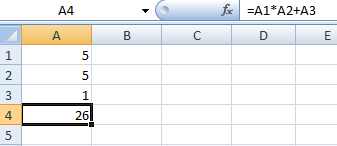In the above example
>> Excel has performed multiplication (A1 * A2) first
>>then, Excel added the value of cell A3 to the result.
Let's see another example,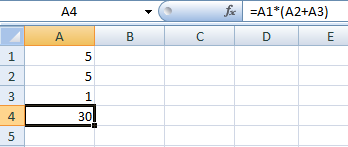In this example
>> Excel have calculated the (A2+A3) first and
>> Then,. It had multiplied the result by the value of cell A1.

### How to Copy & Paste a Formula

When you copy a formula from one cell to another, the excel automatically adjusts the cell references for each value.

For this you have to
• Select the cell containing the formula you want to copy
• Click copy (Ctrl + C) , click on the cell where you want to paste the formula.
• Click paste (Ctrl + V)

Let's learn with example :
>> Enter the formula shown below i.e =A1*(A1+A2)  into cell A4 with any value you want to use.
>> Add value in column C>> Select cell A4, right click, and then click Copy or press (CTRL + C)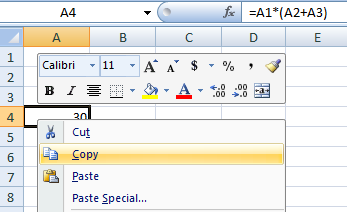>> Now, Select cell C4, right click it
>> Click Paste. (or press CTRL + V).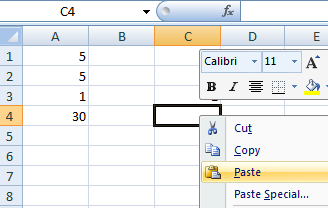>> The Result will be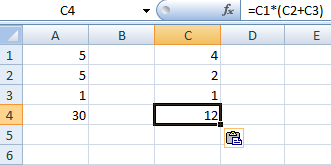There is Another way of copy & paste the formula, wherein you can drag the formula from one cell to another >> You can also drag the formula to cell C4.
>> Select cell A4, click on the lower right corner of cell A4 and drag it to cell C4.
>> This also gives the same result.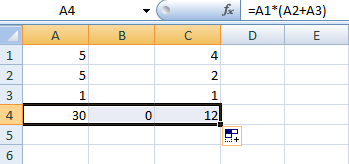** The value of Cell B4 is zero(0) because there are no reference values in Cell B1, B2 & B3.

### How to Insert a Function

Sometimes, you do not want to type formula manually in Excel. This is the high time you can use Insert Function feature to quickly calculate.
>> All function has same structure. For example, SUM(A1:A5). This function is SUM.
>> The brackets part which is called arguments means we give Excel the range A1:A5 as input.
>> This function adds the values in cells A1, A2, A3 ,A4 & A5
Let's learn with following steps
>> Select a cell where you want to insert the Function.
>> Click the Formula tab on the Ribbon, select Insert Function. Insert Function dialog box will appear.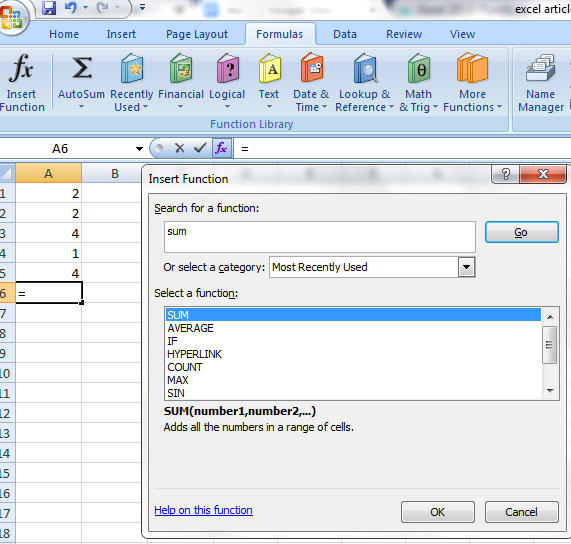>> Search or select a function from list. For example, choose SUM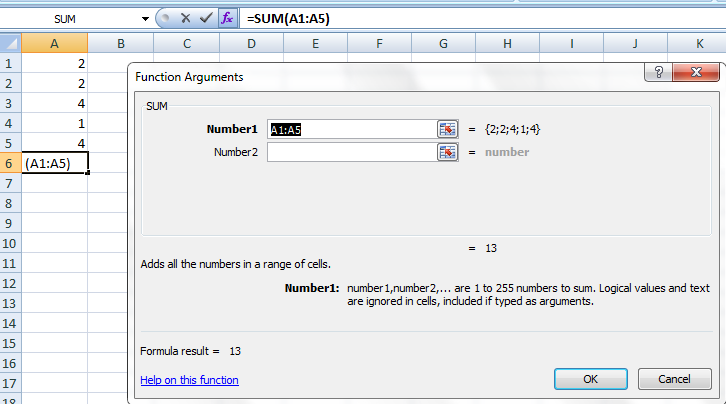>> Click OK and the result will be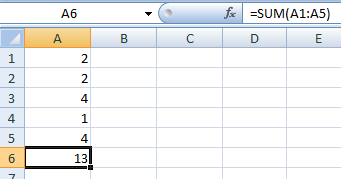>> Likewise you can choose any function from the list.